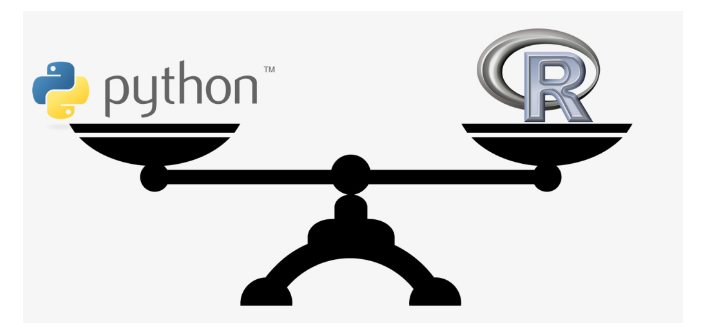## 概述

Scala 和 Excel 是两个极端，对于大多数创业公司而言，我们没有足够多的人手来实现专业化的分工，更多情况下，我们会在 Python 和 R 上花费更多的时间同时完成数据分析（A型）和数据构建（B型）的工作。而许多人也对 Python 和 R 的交叉使用存在疑惑，所以本文将从实践角度对 Python 和 R 中做了一个详细的比较。

## 应用场景对比

### 应用Python的场景

• 网络爬虫/抓取：尽管 rvest 已经让 R 的网络爬虫/抓取变得容易，但 Python 的 beautifulsoup 和 Scrapy 更加成熟、功能更强大，结合django-scrapy我们可以很快的构建一个定制化的爬虫管理系统。

• 连接数据库: R 提供了许多连接数据库的选择，但 Python 只用 sqlachemy 通过ORM的方式，一个包就解决了多种数据库连接的问题，且在生产环境中广泛使用。Python由于支持占位符操作，在拼接SQL语句时也更加方便。

• 内容管理系统：基于Django，Python可以快速通过ORM建立数据库、后台管理系统，而R
中的 Shiny 的鉴权功能暂时还需要付费使用。

### 应用R的场景

• 统计分析: 尽管 Python 里 Scipy、Pandas、statsmodels 提供了一系列统计工具 ，R 本身是专门为统计分析应用建立的，所以拥有更多此类工具。

• 互动式图表/面板: 近来 bokeh、plotly、 intuitics 将 Python 的图形功能扩展到了网页浏览器，甚至我们可以用tornado+d3来进一步定制可视化页面，但 R 的 shiny 和 shiny dashboard 速度更快，所需代码更少。

## 数据流编程对比

1. 参数传递

2. 数据读取

3. 基本数据结构对照

4. 矩阵转化

5. 矩阵计算

6. 数据操作

### 参数传递

Python/R 都可以通过命令行的方式和其他语言做交互，通过命令行而不是直接调用某个类或方法可以更好地降低耦合性，在提高团队协作的效率。

### 数据流编程对比的示例

Python 的 Pandas 中的管道操作
``````  (df
.groupby(['a', 'b', 'c'], as_index=False)
.agg({'d': sum, 'e': mean, 'f', np.std})
.assign(g=lambda x: x.a / x.c)
.query("g > 0.05")
.merge(df2, on='a'))``````
R 的 dplyr 中的管道操作
``````flights %>% group_by(year, month, day) %>%
select(arr_delay, dep_delay)

summarise(

arr = mean(arr_delay, na.rm = TRUE),

dep = mean(dep_delay, na.rm = TRUE)) %>%

filter(arr > 30 | dep > 30)``````

## 数据可视化对比

### 绘制相关性散点图

Python
``````import seaborn as sns
import matplotlib.pyplot as plt
sns.pairplot(nba[["ast", "fg", "trb"]])
plt.show()``````
R
``````library(GGally)
ggpairs(nba[,c("ast", "fg", "trb")])``````

### 绘制聚类效果图

Python
``````from sklearn.cluster import KMeans
kmeans_model = KMeans(n_clusters=5, random_state=1)
good_columns = nba._get_numeric_data().dropna(axis=1)
kmeans_model.fit(good_columns)
labels = kmeans_model.labels_

from sklearn.decomposition import PCA
pca_2 = PCA(2)
plot_columns = pca_2.fit_transform(good_columns)
plt.scatter(x=plot_columns[:,0], y=plot_columns[:,1], c=labels)
plt.show()``````
R
``````library(cluster)
set.seed(1)
isGoodCol <- function(col){
sum(is.na(col)) == 0 && is.numeric(col)
}
goodCols <- sapply(nba, isGoodCol)
clusters <- kmeans(nba[,goodCols], centers=5)
labels <- clusters\$cluster

nba2d <- prcomp(nba[,goodCols], center=TRUE)
twoColumns <- nba2d\$x[,1:2]
clusplot(twoColumns, labels)``````

## 速度对比

Python
``````import numpy as np
xx = np.zeros(100000000)
%timeit xx[:] = 1``````
``````The slowest run took 9.29 times longer than the fastest. This could mean that an intermediate result is being cached
1 loops, best of 3: 111 ms per loop``````
R
``````xx <- rep(0, 100000000)
system.time(xx[] <- 1)``````
``````user  system elapsed
1.326   0.103   1.433``````

## 结论

Python 的 pandas 从 R 中偷师 dataframes，R 中的 rvest 则借鉴了 Python 的 BeautifulSoup，我们可以看出两种语言在一定程度上存在的互补性，通常，我们认为 Python 比 R 在泛型编程上更有优势，而 R 在数据探索、统计分析是一种更高效的独立数据分析工具。所以说，同时学会Python和R这两把刷子才是数据科学的王道。